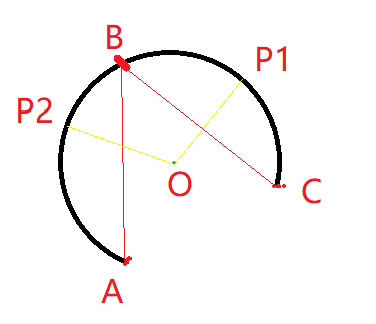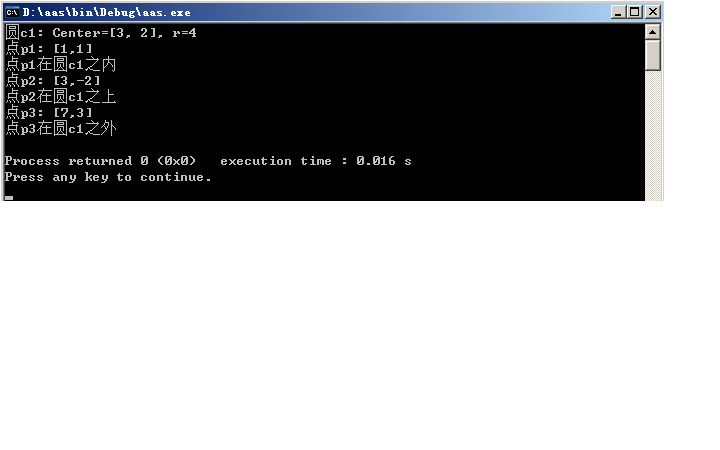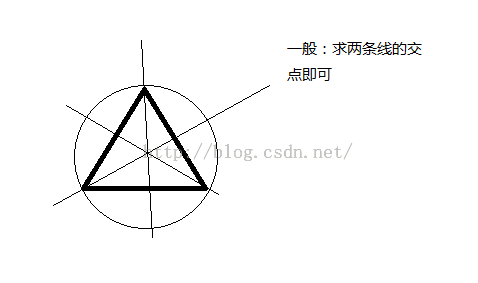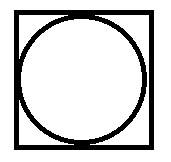• 2021-04-30 11:51:07

## 如何判断点是否在圆弧上

已知一个圆弧三点，A(起点)，B(中点)，C(结束点)。

可以求出圆心、半径红色的分别是圆上的起点、中点、结束点。

1、首先判断圆心到某点P的距离，是否等于半径。

如果不等于半径，则不在圆上


2、连接AB和BC，连接中心点O到点P

如果线段AB或BC其一与OP相交，则点P在圆弧上
反之，点P不在圆弧上


代码实现比较简单，就不贴了

更多相关内容
• 如何计算曲线 y(x) ~y(x)~ y(x) 的曲率，而曲线是由若干离散构成。我的第一反应是根据离散差分得到一阶导数 y′ ~y’~ y′ 和二阶导数 y′′ ~y”~ y′′ ，然后由下式计算 k=∣y′′∣(1+y′2)3/...
• 给定三个，该函数确定通过这三个的中心和半径。 它使用矩阵次要进行分析。 尽管用 R14 编写，但它也可能适用于大多数（旧）版本。 当前版本 2.0，2008 年 3 月 此提交的灵感来自 Peter Bone 于 3-6-2008 ...matlab
• //判断p在圆上、圆内或圆外，返回值：圆内，==0圆上，>0 圆外 protected: double radius; }; //重载运算符“”，使之按规定的形式输出圆的信息 ostream &operator(ostream &output,const Circle &c) { ...
#include <iostream>
#include<Cmath>
using namespace std;
class Point
{
public:
Point(double a=0,double b=0):x(a),y(b) {} //构造函数
double distance(const Point &p) const;	//求距离
friend ostream & operator<<(ostream &,const Point &);//重载运算符“<<”
protected:	  //受保护成员
double x,y;
};

double Point::distance(const Point &p) const	//求距离
{
double dx = x-p.x;
double dy = y-p.y;
return sqrt(dx*dx+dy*dy);
}

ostream & operator<<(ostream &output,const Point &p)
{
output<<"["<<p.x<<","<<p.y<<"]"<<endl;
return output;
}

class Circle:public Point //circle是Point类的公用派生类
{
public:
Circle(double a=0,double b=0,double r=0) :Point(a,b),radius(r) { }; //构造函数
friend ostream &operator<<(ostream &,const Circle &);//重载运算符“<<”
friend int locate(const Point &p, const Circle &c); //判断点p在圆上、圆内或圆外，返回值：<0圆内，==0圆上，>0 圆外
protected:
};

//重载运算符“<<”，使之按规定的形式输出圆的信息
ostream &operator<<(ostream &output,const Circle &c)
{
return output;
}

//判断点p在圆内、圆c内或圆c外
int locate(const Point &p, const Circle &c)
{
const Point cp(c.x,c.y); //圆心
double d = cp.distance(p);
if (abs(d - c.radius) < 1e-7)
return 0;  //相等
return -1;  //圆内
else
return 1;  //圆外
}

int main( )
{
Circle c1(3,2,4);
Point p1(1,1),p2(3,-2),p3(7,3);  //分别位于c1内、上、外

cout<<"圆c1: "<<c1;

cout<<"点p1: "<<p1;
cout<<"点p1在圆c1之"<<((locate(p1, c1)>0)?"外":((locate(p1, c1)<0)?"内":"上"))<<endl;

cout<<"点p2: "<<p2;
cout<<"点p2在圆c1之"<<((locate(p2, c1)>0)?"外":((locate(p2, c1)<0)?"内":"上"))<<endl;

cout<<"点p3: "<<p3;
cout<<"点p3在圆c1之"<<((locate(p3, c1)>0)?"外":((locate(p3, c1)<0)?"内":"上"))<<endl;
return 0;
}


运行结果展开全文• } 判断一个是否位于圆内（圆由三个圆上点确定）。 思路： 1. 圆上任意三个不共线。 2. 圆上三个构成的三角形的垂直平分线交于圆心，即三角形的外接圆。 3. 直线方程的点斜式，y=k(x-x1)+y1。 4. 斜率的...
三角形的外接圆圆心是任意两边的垂直平分线的交点。 三角形外接圆圆心叫外心。
        /// <summary>
/// Returns true if the point (p) lies inside the circumcircle made up by points (p1,p2,p3)
/// </summary>
/// <remarks>
/// NOTE: A point on the edge is inside the circumcircle
/// </remarks>
/// <param name="p">Point to check</param>
/// <param name="p1">First point on circle</param>
/// <param name="p2">Second point on circle</param>
/// <param name="p3">Third point on circle</param>
/// <returns>true if p is inside circle</returns>
private static bool InCircle(Geometry.Point p, Geometry.Point p1, Geometry.Point p2, Geometry.Point p3)
{
//Return TRUE if the point (xp,yp) lies inside the circumcircle
//made up by points (x1,y1) (x2,y2) (x3,y3)
//NOTE: A point on the edge is inside the circumcircle
// 确定三个点是否组成三角形，三点共线则不构成圆
if (System.Math.Abs(p1.Y - p2.Y) < double.Epsilon && System.Math.Abs(p2.Y - p3.Y) < double.Epsilon)
{
//INCIRCUM - F - Points are coincident !!
return false;
}

double m1, m2;
double mx1, mx2;
double my1, my2;
double xc, yc;

if (System.Math.Abs(p2.Y - p1.Y) < double.Epsilon)
{
//计算边P2P3的垂直平分线的斜率
m2 = -(p3.X - p2.X) / (p3.Y - p2.Y);
//计算边P2P3的中点
mx2 = (p2.X + p3.X) * 0.5;
my2 = (p2.Y + p3.Y) * 0.5;
//Calculate CircumCircle center (xc,yc)
xc = (p2.X + p1.X) * 0.5;
// 边P2P3与P1P2边的垂直平分线联立计算交点
// y=xc 与 y=m2(x-mx2)+my2 联立得
yc = m2 * (xc - mx2) + my2;
}
else if (System.Math.Abs(p3.Y - p2.Y) < double.Epsilon)
{
//计算边P1P2的垂直平分线的斜率
m1 = -(p2.X - p1.X) / (p2.Y - p1.Y);
//计算边P1P2的中点
mx1 = (p1.X + p2.X) * 0.5;
my1 = (p1.Y + p2.Y) * 0.5;
//Calculate CircumCircle center (xc,yc)
// 边P2P3与P1P2边的垂直平分线联立计算交点
// y=xc 与 y=m1(x-mx1)+my1 联立得
xc = (p3.X + p2.X) * 0.5;
yc = m1 * (xc - mx1) + my1;
}
else
{
//计算边P1P2和P2P3的垂直平分线的斜率
m1 = -(p2.X - p1.X) / (p2.Y - p1.Y);
m2 = -(p3.X - p2.X) / (p3.Y - p2.Y);
//计算边P1P2和P2P3的中点
mx1 = (p1.X + p2.X) * 0.5;
mx2 = (p2.X + p3.X) * 0.5;
my1 = (p1.Y + p2.Y) * 0.5;
my2 = (p2.Y + p3.Y) * 0.5;
//Calculate CircumCircle center (xc,yc)
// y=m1*(x-mx1)+my1 与 y=m2*(x-mx2)+my2 联立
xc = (m1 * mx1 - m2 * mx2 + my2 - my1) / (m1 - m2);
yc = m1 * (xc - mx1) + my1;
}
//计算圆的半径
double dx = p2.X - xc;
double dy = p2.Y - yc;
double rsqr = dx * dx + dy * dy;

//double r = Math.Sqrt(rsqr); //Circumcircle radius
//计算点到圆心的距离
dx = p.X - xc;
dy = p.Y - yc;
double drsqr = dx * dx + dy * dy;
//判断点到圆心的距离是否小于圆的半径
return (drsqr <= rsqr);
}判断一个点是否位于圆内（圆由三个圆上的点确定）。

思路：

1. 圆上任意三个点不共线。

2. 圆上三个点构成的三角形的垂直平分线交于圆心，即三角形的外接圆。

3. 直线方程的点斜式，y=k(x-x1)+y1。

4. 斜率的计算要考虑垂直于y轴的情况，即y1=y2的情况。

展开全文C# 计算几何
• 这是我通过几篇文章的整合，运用到地图中多个是否在圆内，以及缩小数据范围。实现思路： 1、先根据一个（x纬度,y经度）获取到以半径*2边长的正方形，用来缩小数据范围。 2、获取到正方形内所有的后，然后...

这是我通过几篇文章的整合，运用到地图中多个点是否在圆内，以及缩小数据范围。
实现思路：
1、先根据一个点（x纬度,y经度）获取到以圆半径*2边长的正方形，用来缩小数据范围。2、获取到正方形内所有的点后，然后依次判断数据是否在圆内。
代码如下：

/**
* @Package com.xoxo.arithmetic
* @Description 判断点是否在圆内
* @Author xoxo
* @Date 2018-10-12 17:18
*/
public class PointInMapArithmetic {
private double cx = 0.0D;
private double cy = 0.0D;
private double r = 0.0D;
private static final double EARTH_RADIUS = 6378137.0d;
public PointInMapArithmetic(double x, double y, double r) {
try {
this.cx = x;
this.cy = y;
this.r = r;
} catch (NumberFormatException localNumberFormatException) {
}
}

public boolean isInArea(double x, double y) {
return isInArea(x, y);
}

public boolean isInArea(String x, String y) {
double dis = computeLength(Double.parseDouble(x), Double.parseDouble(y), this.cx, this.cy);
if (dis < this.r) {
return true;
}
return false;
}

public double computeLength(double dwStartX, double dwStartY, double dwEndX, double dwEndY) {
double PI = 3.1415926535898D;
double latRadians1 = dwStartY * (PI / 180.0D);
double latRadians2 = dwEndY * (PI / 180.0D);
double lngRadians = dwStartX * (PI / 180.0D) - dwEndX * (PI / 180.0D);
double f = 2.0D * Math.asin(Math.sqrt(Math.pow(Math.sin(latRadians / 2.0D), 2.0D)
}

/**
* <p>Description: 计算两点之间距离，获取一个圆半径为边长*2的正方形面积，一般用于减少大量不在圆内范围的数据</p>
* @param dis 距离
* @param lng 起始经度
* @param lat 起始纬度
* @return p[yMin][yMax][xMin][xMax]正方形范围
*/
public static double[] getPoiRange(double dis, double lng, double lat) {
double[] poi = new double;

double lng1 = (Math.PI / 180) * lng;
double lng2 = (Math.PI / 180) * lng;
double lat1 = (Math.PI / 180) * lat;
double lat2 = (Math.PI / 180) * lat;

// 角度
double theta = dis / EARTH_RADIUS;

// 维度相同，反算经度
double lngVal = Math.cos(theta) - Math.sin(lat1) * Math.sin(lat2);
double lngThetaVal = lngVal / (Math.cos(lat1) * Math.cos(lat2));
double elng1 = (-Math.acos(lngThetaVal) + lng1) / (Math.PI / 180);
double elng2 = (Math.acos(lngThetaVal) + lng1) / (Math.PI / 180);

//LogLIB.LogRun.info("elng1=" + UtilTools.format(elng1) + " elng2=" + UtilTools.format(elng2));

// 经度相同，反算维度（根据辅助角公式计算 asinx + bcosx）
// theta = a * Math.sin(lat2) + b * Math.cos(lat2) = Math.sqrt(a平方 + b平方)sin(x + arctan(b/a))
double a = Math.sin(lat1);
double b = Math.cos(lat1) * Math.cos(lng2 - lng1);
double sqrt = Math.sqrt(Math.pow(a, 2) + Math.pow(b, 2));
double latTheteVal = Math.cos(theta) / sqrt;
double elat1 = (Math.asin(latTheteVal) - Math.atan(b/a)) / (Math.PI / 180);
double elat2 = (Math.asin(-latTheteVal) - Math.atan(b/a)) / (Math.PI / 180) + 180;

//LogLIB.LogRun.info("elat1=" + UtilTools.format(elat1) + " elat2=" + UtilTools.format(elat2));

poi = elng1;
poi = elng2;
poi = elat1;
poi = elat2;

return poi;
}
public static void main(String[] args) {
PointInMapArithmetic mapArithmetic = new PointInMapArithmetic(116.334616, 39.984912, 1000);
System.out.println(mapArithmetic.isInArea("116.324", "39.984912"));
double[] poiRange = getPoiRange(1000.0,39.984912,116.334616);
for (double p:
poiRange) {
System.out.println(p);
}
}
}

展开全文地图算法
• 匿名用户1级2016-09-02 回答问题：已知圆上的两坐标和半径，求圆心。数学分析：这个题目，涉及到简单的数学问题，但是计算比较繁琐。假设已知圆上的两坐标分别为N(X1，Y1)和M(X2，Y2)，半径为R，圆心坐标为o(a,b...
• 平面几何中，3可以确定唯一的，5可以确定唯一的椭圆，本文MATLAB中输入5个，使用无边界多元优化函数确定出唯一的椭圆，用ezplot画出，求出椭圆中心，最后给出了完整代码.
• 通过拟合椭圆的准来检测椭圆的中心，利用椭圆外切矩形的几何性质来确定椭圆的长短轴位置及旋转角度。为了去除非标志的干扰边缘影响，进一步构造验证参数，并结合聚类算法，最终提取有效的标志类。仿真与实物...
• 早年写的面试题，已知圆上任意三点求圆心半径 c++实现，有兴趣可以一观！ 不考虑特殊情况（代码中有用到除法 忽略分母不能为0情况）c++
• 1.根据圆上的两个和半径获得两个圆的算法 #define MAXCOM_F(a, b) ((a)-(b)&gt;0.00001) ? true : false QVector&lt;QRectF&gt; EICWidget::getEllipseCoors(const QPointF &amp;r1, const ...算法
• 1、已知圆上几个点确定空间圆 2、绘制出圆和圆心 3、输入数据直接调用即可 4、有详细算法原理解释文档代码
• ## 圆上点的坐标

万次阅读 2018-10-08 10:39:09
圆上点的坐标需要已知的条件：圆心、半径、角度 假设圆心:o (x0,y0) 半径:r 角度:angle (角度是相对于图中红点位置而言，逆时针为负数，顺时针为正)   计算公式：  p2 (x1,y1), 其中angle = 30   x1 ...
• 开发路线类应用绘制曲线的时候，遇到了一个问题就是：已知曲线起点和终点的坐标、曲率半径及前进方向四个条件绘制曲线。而封装好的绘制曲线的函数是根据极坐标方法绘制的，因此需要提供四个参数。 【1】...算法 C# CAD二次开发
• 策划给了个需求，给定一个圆环，内径r1，外径r2，要圆环内随机产生这些位置生成法术场。那么如何圆环内随机产生这些呢？ 在圆内随机生成 首先考虑在圆内生成随机。 最简单的方法是一个R*R的...
• ## 求圆上点的坐标

万次阅读 2016-09-17 00:16:21
圆上点的坐标需要已知的条件：圆心、半径、角度 假设圆心:o (x0,y0) 半径:r 角度:angle (角度是相对于图中红点位置而言，逆时针为负数，顺时针为正) 计算公式： p2 (x1,y1), 其中angle = 30 ...qt 公式
• 牛客题目链接 .../**遇到此类型的题目就是先确定圆心再去判断其他店是否在圆上或者圆内**/ #include <map> #include <queue> #include <string> #include<iostream> #include&c++ 数学
•c++
• 平面，如果已知△P0P1P2\triangle P_0 P_1 P_2的三个顶点坐标P0(x0,y0), P1(x1,y1), P2(x2,y2)P_0(x_0, y_0),\space P_1(x_1,y_1),\space P_2(x_2,y_2)和另一点PP的坐标(x,y)(x,y)，要判断P是否△P0P1P2\...几何算法
• // i： 圆上点的个数，默认15个，建议73个 countCircle(t, e, i) { for( var r = t / 6378137, n = [e, e], o = [this.numberToRadius(n), this.numberToRadius(n)], s = ((i = i || 15), []), a ...javascript vue.js
• 首先可以先确定一个的申明方式，后续可以通过确定圆心。 #ifndef _POINT_H #define _POINT_H class Point {  private:  int m_x;  int m_y;  public:  void setxy(int x,int y);  int getdistance(Poi....
• 判断一个点在曲线的理论依据是什么呢？前面已经说了，素域Fp的椭圆曲线E由（仿射）方程y2＝x3＋ax＋b决定，所以Jacobi射影P＝(X,Y,Z)曲线就看这个是不是满足射影方程y2＝x3＋axz4＋bz6。带入P分别...openssl
• 苏教版九年级上册数学[的有关概念及确定—知识整理及重点题型梳理].doc
• 通过输入任意三点空间坐标自动判断三点是否一条直线，若不则求三点外接的圆心位置和半径，计算精度可调节。解决了“知三点求圆心半径”程序对于某些无法计算的缺陷。
• //的方程：x^2+y^2=r^2 //由于默认y=sqrt(r^2-x^2)是double类型 //判断y是不是整数 import java.util.*; public class Test { /** * @param args */ static int count=0; public static void main...
• Java：定义一个未知，判断未知是否在圆内。如果在圆内，输出true，否则输出false。题目要求设计思路代码类方法运行结果 题目要求 设计思路 设计思路根据题目要求： 1.设计一个类代表二维空间的一个...java
• 那么这个圆的方程可以写为：这个圆上随便取三个，设这三个的坐标分别是那么有：公式(1)(2)相减，(1)(3)相减之后经过化简可以得到：有唯一解的条件是系数行列式不为 0 ：简单变变型也就是：这样写几何含义就...
• 设计一个圆形类（Circle），和一个类（Point），计算和圆的关系（圆上、圆外、圆内）。 例程如下： #include<iostream> #include<string> using namespace std; //一个类（Point） class ...c++
• 加强记忆。。。 圆点坐标：(x0,y0) 半径：r 角度：angel 则上任一点为：（x1,y1） x1 = x0 + r * cos(angel * PI /180 ) y1 = y0 + r * sin(angel * PI /180 )
• 本文是利用MATLAB实现长度为L（L>2倍波长） 的传输线沿线...已知负载阻抗和特性阻抗的情况下，阻抗圆图上确定负载阻抗，通过MATLAB实现该点在等反射系数圆上的转动，最终得出输入阻抗值。附录给出MATLAB源代码。...